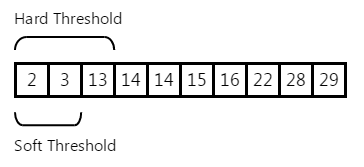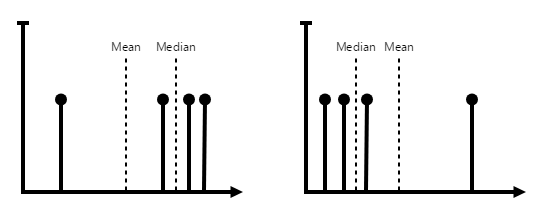## Candidate Selection Using Iterative Soft-Thresholding

Wednesday, June 21, 2017

This article describes one way to use soft-thresholding to select the statistically best candidates from a sorted list. This algorithm was introduced to me as an alternative to setting a hard threshold, i.e. selecting a fixed number of the best candidates. Using an iterative soft-thresholding algorithm a variable number of candidates can be selected depending on the distribution of the values.

In the following example the best candidates are selected from a sorted list. Setting a hard threshold of three will of course always select the top three candidates. However, it is clear from looking at the distribution of the values that only the top two could be considered as candidates. This soft-thresholding algorithm allows us to select just those candidates.### How the algorithm works

In each iteration the algorithm compares the mean and the median of the values remaining in the list. Any values higher than the minimum of the mean and median are discarded. The process is repeated until exit conditions are satisfied or until there is only one value remaining.### Sample code

The following sample python code is a simple example to demonstrate how iterative soft-thresholding can be implemented. The sorted list values are randomly generated on each execution of the script. Executing a number of times shows how the number of selected candidates varies based on the distribution.

``````import numpy as np
import numpy.random as npr

# The maximum number of candidates to select
max_candidates = 3
# The sorted list
sorted_list = sorted(npr.random_integers(1, 100, size=25))
print("Sorted list of candidates:", sorted_list)

while len(sorted_list) > 1:
# Output remaining candidates
print("Remaining candidates:", len(sorted_list))

# Calculate the mean, median and standard deviation of the list
mean = np.mean(sorted_list)
median = np.median(sorted_list)
std = np.std(sorted_list)

# If the standard deviation is zero the list values are identical
if std == 0:
sorted_list = sorted_list[:max_candidates]
break

# Stop iterating if the exit conditions for the distribution are met
if (abs(mean - median) < 0.1 * max(mean, median)) & (std < 0.5 * mean):
if len(sorted_list) <= max_candidates:
break

# Remove any values less or equal to the minimum of the mean and median
sorted_list = [_ for _ in sorted_list if _ <= min(mean, median)]

# Results after soft threshold iterations
print("=" * 24)
print("Selected candidates:", sorted_list)``````

One candidate is selected:

``````~\$ python soft_thresholding.py
Sorted list of candidates: [2, 10, 11, 20, 22, 23, 27, 29, 35, 39, 43, 44, 49, 57, 58, 61, 65, 66, 68, 83, 83, 91, 94, 94, 99]
Remaining candidates: 25
Remaining candidates: 13
Remaining candidates: 7
Remaining candidates: 3
========================
Selected candidates: ``````

Two candidates are selected:

``````~\$ python soft_thresholding.py
Sorted list of candidates: [1, 2, 11, 12, 12, 27, 32, 34, 35, 37, 38, 44, 46, 48, 50, 59, 60, 60, 62, 71, 71, 75, 77, 80, 91]
Remaining candidates: 25
Remaining candidates: 12
Remaining candidates: 5
Remaining candidates: 2
========================
Selected candidates: [1, 2]``````

Three candidates are selected:

``````~\$ python soft_thresholding.py
Sorted list of candidates: [2, 3, 4, 5, 5, 6, 12, 12, 16, 17, 20, 21, 26, 27, 32, 34, 41, 53, 55, 58, 59, 61, 72, 86, 96]
Remaining candidates: 25
Remaining candidates: 13
Remaining candidates: 6
Remaining candidates: 3
========================
Selected candidates: [2, 3, 4]``````

### Fine tuning

The maximum number of candidates can be modified in the sample code. The output of the algorithm will be any number of candidates up to this value.

``max_candidates = 3``

The algorithm will continue to iterate until the exit conditions are satisfied. These can be fine tuned to be less or more sensitive. In general, if the candidates are very close in value then we want to stop iterating because all of them will be good potential candidates. If the distribution is sparse then we want to keep iterating.

These are the exit conditions for asymmetrical and symmetrical distributions in the sample code.

``abs(mean - median) < 0.1 * max(mean, median)``
``std < 0.5 * mean``

The fixed values of `0.1` and `0.5` allow the algorithm to be tuned. Decreasing these values will make the exit condition less sensitive and the algorithm will keep iterating. Increasing the value will cause the algorithm to exit sooner.